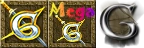This article documents Glest, GAE, and MegaGlest This is noted in the colored backgrounds, which denote lines as being engine specific. See the key below.[Editing help]
v  d  e

Splash particle systems are most commonly used to create special effects from a unit being attacked.

## XML

GAE Only
GAE and MegaGlest Only
```<?xml version="1.0" standalone="yes"?>
<splash-particle-system>
<texture value="true" path=".../texture_name.bmp" luminance="true" />
<model value="true" path=".../model_name.g3d" />
<mode value="normal" />
<offset x="0" y="0" z="0" />
<color red="0.0" green="0.0" blue="1.0" alpha="0.5" />
<color-no-energy red="1.0" green="1.0" blue="1.0" alpha="0.0" />
<size value="1" />
<size-no-energy value="2" />
<speed value="5" />
<gravity value="0" />
<emission-rate value="20" />
<energy-max value="30" />
<energy-var value="10" />
<!-- Start GAE Only -->
<blend-mode src="src_alpha" dest="one" />
<!-- End GAE Only -->
</splash-particle-system>
```

## Documentation

### texture

Particles can use textures to shape how the particles should look. If luminance is turned on, the image file used as a texture will simply work to define the intensity of the colors. If luminance is turned off, the colors in the image file will be used in the particle. The path is the path to the image.

### model

If true, the path to the model which will be used as the attack projectile. Animated models will only show the last frame.

### primitive

Particles have two methods of creation: either quad (renders a set of four particles in a dot style) or line (creates solid lines). Quad is the most commonly used, as it creates the fuzzy style of particles that would be used for flames, explosions, smoke, etc, while lines are generally only used for projectiles such as an arrow. Note that you cannot have more than one particle effect linked to a projectile or splash (though you can for unit particle effects) yet, so it is not possible to mix particles (such as a line and flaming particles for a flaming arrow).

### mode

Particles have two modes of rendering, either normal which uses colors, or as black which allows the usage of black particles. If not specified, it defaults to normal.

### color and color-no-energy

If luminance is off, the colors of the texture will be used to color the particles. If luminance is on, the colors defined in the XML are used, though the intensity and shape will be defined by the texture. There are two colors values, the first which is the color at the start of the particle's life, and the second which defines the color when the particle is dead. Thus, the particles can transition in color. Colors are rendered by mixing red, green, and blue, as well as you can set the alpha channel. Each parameter is from 0 - 1, where 1 means there is 100% of that color. With the alpha, 1 would mean the color is almost completely transparent while 0 would be completely opaque.

### size and size-no-energy

While the shape - and thus some degree of the size - is defined by the texture, the size is also affected by the size parameter passed into the XML, which will define the size in game units. Like the color, there are two size values that define the starting and ending sizes. As a result, the particles can seem to shrink or grow over their life.

### gravity

Glest has a simple gravity system which can also cause particles to sink downwards - or float upwards - over their life. The value is the degree of sink - or in the case of a negative number, the degree of rise.

### emission-rate

The number of individual particles that are emitted.

### energy-max

The starting energy of the particles.

### energy-var

A variance to add or subtract from energy-max to make particles more random.

The rate at which the particles disappear. A lower number will have particles lasting longer, while a higher number will have shorter lasting particles.

### blend-mode

An optional GAE only value that sets the blend mode for the particle system. For details, see the OpenGL reference for the glBlendFunc() function.

Legal values are:

• zero
• one
• src_color
• one_minus_src_color
• dst_color
• one_minus_dst_color
• src_alpha
• one_minus_src_alpha
• dst_alpha
• one_minus_dst_alpha
• constant_color
• one_minus_constant_color
• constant_alpha
• one_minus_constant_alpha
• src_alpha_saturate (cannot be used for dest)

The value is optional, and if not specified, the default is the same as classic Glest behavior, equivalent to <blend-mode src="src_alpha" dest="one"/> However, to enable particles to contain dark colors (and not have the brightness used as an alpha value), you could use <blend-mode src="src_alpha" dest="one_minus_src_alpha"/>

### blend-equation

A GAE only optional value that sets the blend equation for the particle system. For details, see the OpenGL reference for the glBlendEquation() function.

Legal values are: